## General Chemistry

In a chemical reaction, stoichiometry shows the mole-ratio of the reactants and products based on the coefficients which are written before them. It allows calculating the amount of any component of a chemical reaction if one of them is given using the mole method.

A chemical equation can be interpreted at molecular and molar levels. For example, the following equation shows the combustion reaction of ethanol (C2H5OH) producing carbon dioxide (CO2) and water (H2O):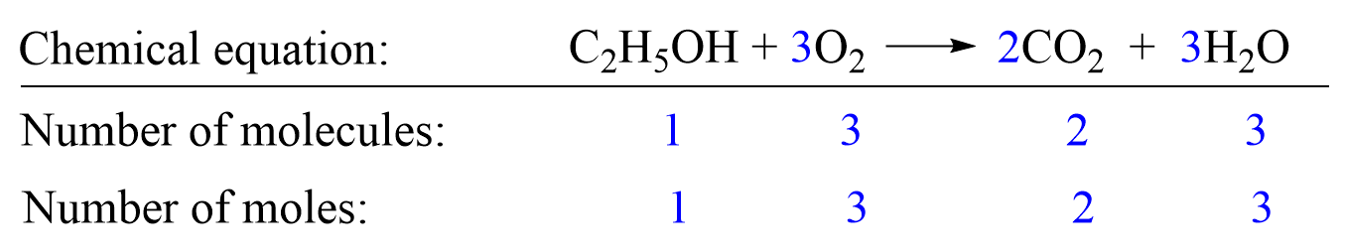Molar level: The coefficients in blue indicate, for example, that 1 mole of C2H5OH reacts with 3 moles of O2 producing 2 moles of CO2 and 3 moles of H2O.

At the molecular level, it tells us that 1 molecule of C2H5OH reacts with 3 molecules of O2 producing 2 molecules of CO2 and 3 molecules of H2O.

So, the coefficients show the ratio of the reaction components which is like the recipe of the reaction. However, we can have any number of moles of reactants, and, using stoichiometry (the ratio of the components), we can calculate how much products can be obtained.

For example, let’s determine how much CO2 will be formed from 3.0 moles of C2H5OH by the following combustion reaction:

C2H5OH + 3O2   →   2CO2 + 3H2O

The first thing is to set up the conversion factors correlating the two components – the one with the known amount and the target which we need to determine. This is done using the coefficients in the chemical equation which indicate that 1 mole of C2H5OH produces 2 moles of CO2. Conversion factors are the two ratio fractions of these coefficients:

C2H5OH + 3O2   →   2CO2 + 3H2O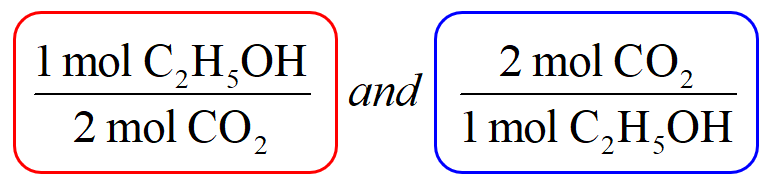Now, because you are asked to determine the amount of CO2, pick the conversion factor such that C2H5OH is the denominator and can be canceled:Next, write the moles of C2H5OH that are reacted (not the coefficient) and multiply it with this conversion factor: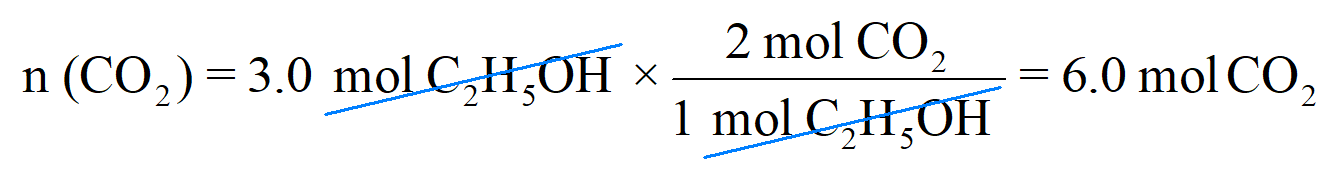Therefore, 3.0 mol C2H5OH will produce 6 mol CO2, and this makes sense because the stoichiometry of the reaction tells us that there will always be two times more CO2 produced from a given amount of C2H5OH. So, if it was, for example, 5 mol C2H5OH, 10 mol CO2 would have been produced.

Let’s look at another example, where we need to determine the amount of a reactant required to produce certain moles of a product. Remember, as long as you know the quantity of one component in the reaction, whether it is a reactant or product, you can determine the amount of any other component.

For example: How many moles of N2 must be used to produce 10 moles of NH3 according to the following chemical equation?

3H2 + N2 → 2NH3

Step 1. Write a conversion factor between N2 and NH3 such that NH3 is on the bottom and can be canceled out: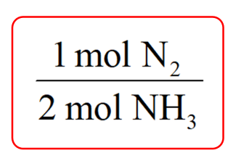Step 2. Write the moles of ammonia (10 mol) and multiply it by the conversion factor: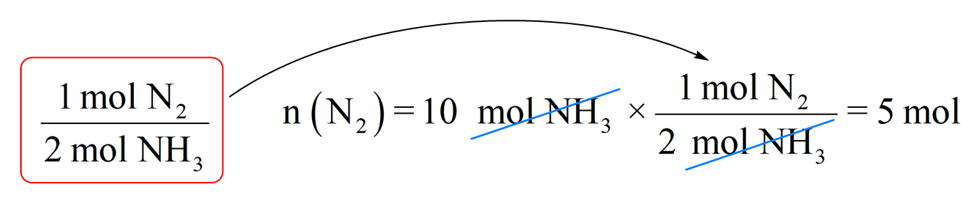So, we will need 5 mol NH3 to produce 10 mol NH3 and this is consistent with the chemical equation which indicates that the amount of nitrogen required to produce ammonia is always two times less because of the 1:2 mole ratio.

# Mass Calculations using Reaction Stoichiometry

Sometimes, or perhaps more often than not, the amounts of reaction components are given in grams or any other units. Remember, you will always need to convert them to moles first and then use the conversion factors to do the necessary calculations. This is, again, because chemical equations tell us the mole-ratio of the components by based on their coefficients.

For example: How many grams of Ba(NO3)2 can be prepared by reacting 16.5 g of HNO3 with an excess of Ba(OH)2?

Ba(OH)2 + 2HNO3  →  Ba(NO3)2 + 2H2O

The plan would be to calculate the moles of HNO3, then use the mole-ratio to determine the moles of Ba(NO3)2, and finally, convert the moles to the mass of Ba(NO3)2: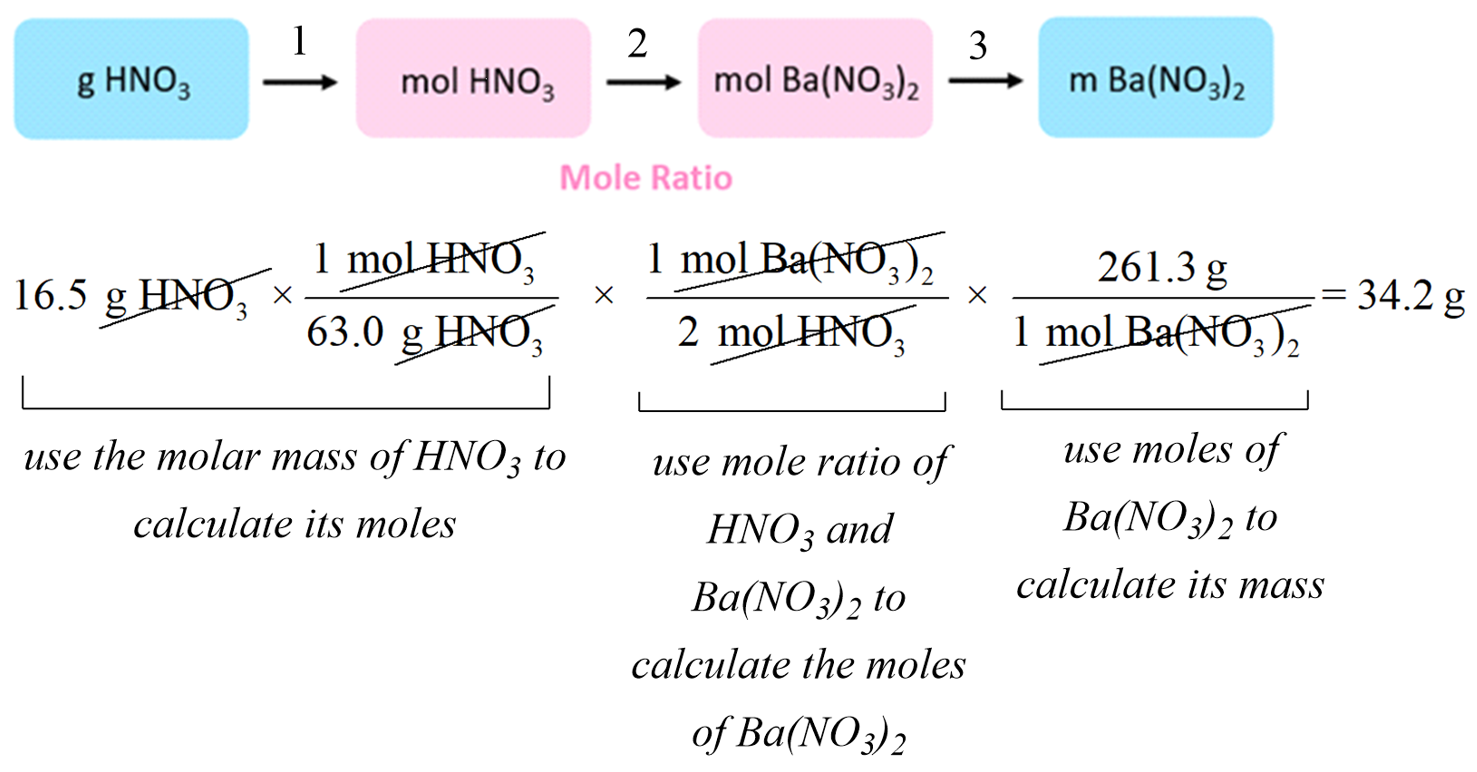You can also do this step-by-step to better follow the entire calculation: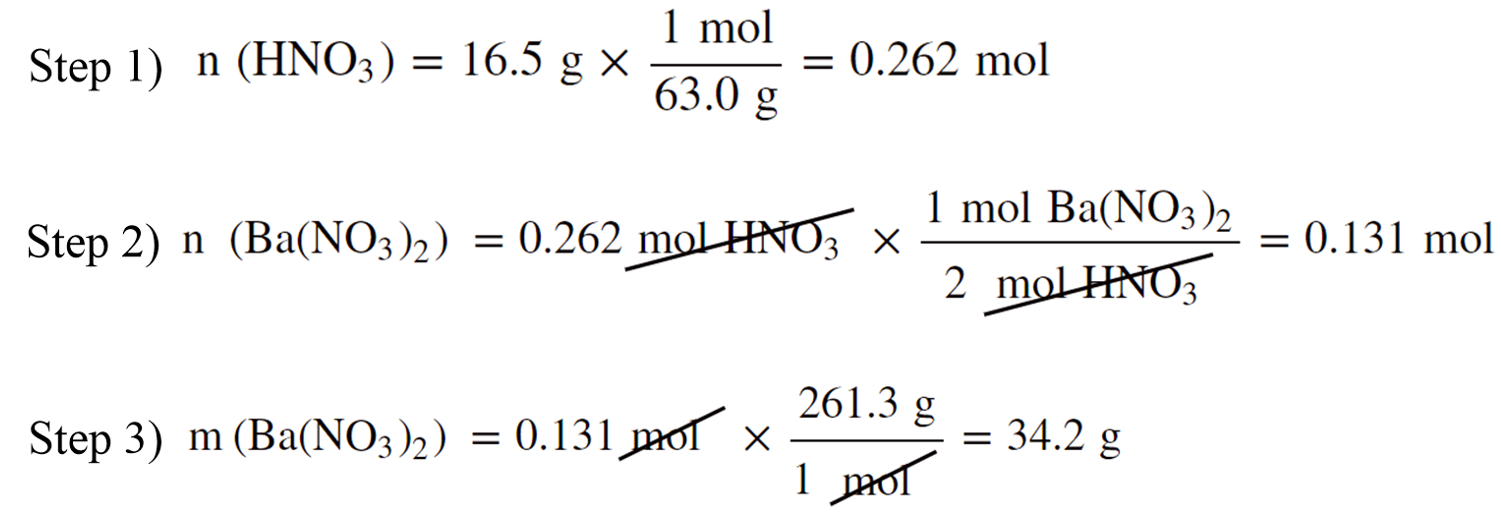#### Practice

1.

Consider the balanced equation:

C5H12 + 8 O2 → 5CO2 + 6H2O

Complete the table showing the appropriate number of moles of reactants and products.

This content is available to registered users only. Click here to Register! By joining Chemistry Steps, you will gain instant access to the answers and solutions for all the Practice Problems and the powerful set of General Chemistry 1 and 2 Summary Study Guides.
Solution
This content is available to registered users only. Click here to Register! By joining Chemistry Steps, you will gain instant access to the answers and solutions for all the Practice Problems and the powerful set of General Chemistry 1 and 2 Summary Study Guides.
2.

How many grams of CO2 and H2O are produced from the combustion of 220. g of propane (C3H8)?

C3H8(g) + 5O2(g) → 3CO2(g) + 4H2O(g)

This content is available to registered users only. Click here to Register! By joining Chemistry Steps, you will gain instant access to the answers and solutions for all the Practice Problems and the powerful set of General Chemistry 1 and 2 Summary Study Guides.
Solution
This content is available to registered users only. Click here to Register! By joining Chemistry Steps, you will gain instant access to the answers and solutions for all the Practice Problems and the powerful set of General Chemistry 1 and 2 Summary Study Guides.
3.

How many grams of CaCl2 can be produced from 65.0 g of Ca(OH)2 according to the following reaction,

Ca(OH)2 + 2HCl → CaCl2 + 2H2O

This content is available to registered users only. Click here to Register! By joining Chemistry Steps, you will gain instant access to the answers and solutions for all the Practice Problems and the powerful set of General Chemistry 1 and 2 Summary Study Guides.
Solution
This content is available to registered users only. Click here to Register! By joining Chemistry Steps, you will gain instant access to the answers and solutions for all the Practice Problems and the powerful set of General Chemistry 1 and 2 Summary Study Guides.
4.

How many moles of oxygen are formed when 75.0 g of Cu(NO3)2 decomposes according to the following reaction?

2Cu(NO3)2  → 2CuO + 4NO2 + O2

This content is available to registered users only. Click here to Register! By joining Chemistry Steps, you will gain instant access to the answers and solutions for all the Practice Problems and the powerful set of General Chemistry 1 and 2 Summary Study Guides.
Solution
This content is available to registered users only. Click here to Register! By joining Chemistry Steps, you will gain instant access to the answers and solutions for all the Practice Problems and the powerful set of General Chemistry 1 and 2 Summary Study Guides.
5.

How many grams of MnClcan be prepared from 52.1 grams of MnO2?

MnO2 + 4HCl → MnCl+ Cl2 + 2H2O

This content is available to registered users only. Click here to Register! By joining Chemistry Steps, you will gain instant access to the answers and solutions for all the Practice Problems and the powerful set of General Chemistry 1 and 2 Summary Study Guides.
Solution
This content is available to registered users only. Click here to Register! By joining Chemistry Steps, you will gain instant access to the answers and solutions for all the Practice Problems and the powerful set of General Chemistry 1 and 2 Summary Study Guides.
6.

Determine the mass of oxygen that is formed when an 18.3-g sample of potassium chlorate is decomposed according to the following equation:

2KClO3(s) → 2KCl(s) + 3O2(g).

This content is available to registered users only. Click here to Register! By joining Chemistry Steps, you will gain instant access to the answers and solutions for all the Practice Problems and the powerful set of General Chemistry 1 and 2 Summary Study Guides.
Solution
This content is available to registered users only. Click here to Register! By joining Chemistry Steps, you will gain instant access to the answers and solutions for all the Practice Problems and the powerful set of General Chemistry 1 and 2 Summary Study Guides.
7.

How many grams of H2O will be formed when 48.0 grams H2 are mixed with excess hydrogen gas?

2H2 + O2 → 2H2O

This content is available to registered users only. Click here to Register! By joining Chemistry Steps, you will gain instant access to the answers and solutions for all the Practice Problems and the powerful set of General Chemistry 1 and 2 Summary Study Guides.
Solution
This content is available to registered users only. Click here to Register! By joining Chemistry Steps, you will gain instant access to the answers and solutions for all the Practice Problems and the powerful set of General Chemistry 1 and 2 Summary Study Guides.
8.

Consider the chlorination reaction of methane (CH4):

CH4(g) + 4Cl2(g) → CCl4(g) + 4HCl(g)

How many moles of CHwere used in the reaction if 51.9 g of CCl4 were obtained?

This content is available to registered users only. Click here to Register! By joining Chemistry Steps, you will gain instant access to the answers and solutions for all the Practice Problems and the powerful set of General Chemistry 1 and 2 Summary Study Guides.
Solution
This content is available to registered users only. Click here to Register! By joining Chemistry Steps, you will gain instant access to the answers and solutions for all the Practice Problems and the powerful set of General Chemistry 1 and 2 Summary Study Guides.
9.

How many grams of Ba(NO3)2 can be produced by reacting 16.5 g of HNO3 with an excess of Ba(OH)2?

This content is available to registered users only. Click here to Register! By joining Chemistry Steps, you will gain instant access to the answers and solutions for all the Practice Problems and the powerful set of General Chemistry 1 and 2 Summary Study Guides.
Solution
This content is available to registered users only. Click here to Register! By joining Chemistry Steps, you will gain instant access to the answers and solutions for all the Practice Problems and the powerful set of General Chemistry 1 and 2 Summary Study Guides.
10.

Ethanol can be obtained by fermentation – a complex chemical process breaking down glucose to ethanol and carbon dioxide.

C6H12O6   →    2C2H5OH   +    2CO2
glucose                   ethanol

How many mL of ethanol (d =0.789 g/mL) can be obtained by this process starting with 286 g of glucose?

This content is available to registered users only. Click here to Register! By joining Chemistry Steps, you will gain instant access to the answers and solutions for all the Practice Problems and the powerful set of General Chemistry 1 and 2 Summary Study Guides.
Solution
This content is available to registered users only. Click here to Register! By joining Chemistry Steps, you will gain instant access to the answers and solutions for all the Practice Problems and the powerful set of General Chemistry 1 and 2 Summary Study Guides.
11.

36.0 g of butane (C4H10) was burned in an excess of oxygen and the resulting carbon dioxide (CO2) was collected in a sealed vessel.

2C4H10 + 13O2 → 8CO2 + 10H2O

How many grams of LiOH will be necessary to consume all the CO2 from the first reaction?

2LiOH + CO2 → Li2CO3 + H2O

This content is available to registered users only. Click here to Register! By joining Chemistry Steps, you will gain instant access to the answers and solutions for all the Practice Problems and the powerful set of General Chemistry 1 and 2 Summary Study Guides.
Solution
This content is available to registered users only. Click here to Register! By joining Chemistry Steps, you will gain instant access to the answers and solutions for all the Practice Problems and the powerful set of General Chemistry 1 and 2 Summary Study Guides.

### 5 thoughts on “Stoichiometry of Chemical Reactions”

1.Hi, for #8 in the practice questions. The question asks for the mass of CCl4, but the work was only done to solve for the moles of CCl4.

•Oh, thanks. The question was supposed to be rephrased.

•No problem!

2.•